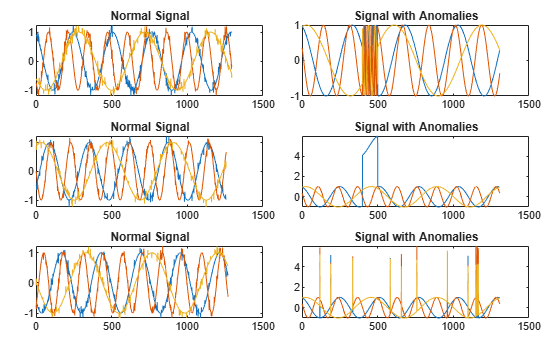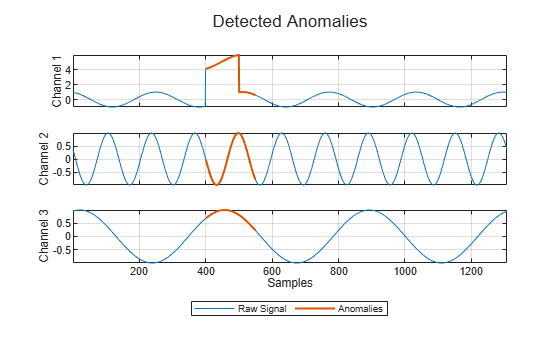# updateDetector

Update settings of trained detector and recompute detection threshold

Since R2023a

## Syntax

``updateDetector(d,data,Name=Value)``

## Description

example

````updateDetector(d,data,Name=Value)` updates the threshold and window settings of the detector `d`, then recomputes the detection threshold. For any name-value argument not specified, the function uses the current value of the corresponding parameter in the detector. NoteWhen `ThresholdMethod` is set to `"manual"`, you do not need to specify input signal data set `data`. ```

## Examples

collapse all

Load a convolutional anomaly detector trained with three-channel sinusoidal signals. Display the model, threshold, and window properties of the detector.

```load sineWaveAnomalyDetector D```
```D = deepSignalAnomalyDetectorCNN with properties: IsTrained: 1 NumChannels: 3 Model Information ModelType: 'conv' FilterSize: 8 NumFilters: 32 NumDownsampleLayers: 2 DownsampleFactor: 2 DropoutProbability: 0.2000 Threshold Information Threshold: 0.0510 ThresholdMethod: 'contaminationFraction' ThresholdParameter: 0.0100 Window Information WindowLength: 1 OverlapLength: 'auto' WindowLossAggregation: 'mean' ```

Load the file `sineWaveAnomalyData.mat`, which contains two sets of synthetic three-channel sinusoidal signals.

• `sineWaveNormal` contains the 10 sinusoids used to train the convolutional anomaly detector. Each signal has a series of small-amplitude impact-like imperfections but otherwise has stable amplitude and frequency.

• `sineWaveAbnormal` contains three signals of similar length and amplitude to the training data. One of the signals has an abrupt, finite-time change in frequency. Another signal has a finite-duration amplitude change in one of its channels. A third has random spikes in each channel.

Plot three normal signals and the three signals with anomalies.

```load sineWaveAnomalyData tiledlayout(3,2,TileSpacing="compact",Padding="compact") rnd = randperm(length(sineWaveNormal)); for kj = 1:length(sineWaveAbnormal) nexttile plot(sineWaveNormal{rnd(kj)}) title("Normal Signal") nexttile plot(sineWaveAbnormal{kj}) title("Signal with Anomalies") end```Update the anomaly detector using the training data.

• Specify the regions to be labeled as anomalies as non-overlapping 50-sample windows.

• Specify that the function compute the detection threshold as the mean window loss measured over the entire training data set and multiplied by `20`.

```updateDetector(D,sineWaveNormal, ... WindowLength=50,OverlapLength=0, ... ThresholdMethod="mean",ThresholdParameter=20)```

Plot the second test signal and annotate the anomalies found by the updated detector.

`plotAnomalies(D,sineWaveAbnormal{2})`## Input Arguments

collapse all

Anomaly detector, specified as a `deepSignalAnomalyDetectorCNN` or `deepSignalAnomalyDetectorLSTM` object. Use the `deepSignalAnomalyDetector` function to create `d`.

Signal data set, specified as one of these:

• Nc-column matrix — A single multichannel signal observation (M = 1), where Nc is equal to the value of the `NumChannels` property of the detector.

• M-element cell array — M multichannel signal observations, where each cell contains an Nc-column matrix.

• Timetable — A single multichannel signal observation, contained in a MATLAB® timetable. The timetable must contain increasing, uniformly-sampled, and finite values. The timetable can have:

• A single variable containing an Nc-column matrix, where each column corresponds to a signal channel.

• Nc variables, where each variable contains a vector that corresponds to a signal channel.

• Datastore — A `signalDatastore`, `audioDatastore` (Audio Toolbox), or `arrayDatastore` object. The detector uses the `readall` function to read all the signal observations contained in the datastore at once. You can also use a `CombinedDatastore` or `TransformedDatastore` object containing any of the supported datastores.

### Name-Value Arguments

Specify optional pairs of arguments as `Name1=Value1,...,NameN=ValueN`, where `Name` is the argument name and `Value` is the corresponding value. Name-value arguments must appear after other arguments, but the order of the pairs does not matter.

Example: `MiniBatchSize=64,ExecutionEnvironment="cpu"` instructs the function to use a mini-batch size of 64 and use the computer CPU to detect anomalies.

Window length of each signal segment, specified as a positive integer or as `"fullSignal"`.

• If you specify `WindowLength` as an integer, the detector divides each input signal into segments. The length of each segment is equal to the specified value in samples.

• If you specify `WindowLength` as `"fullSignal"`, the detector treats each input signal as a single segment.

Data Types: `single` | `double` | `int8` | `int16` | `int32` | `int64` | `uint8` | `uint16` | `uint32` | `uint64` | `char` | `string`

Number of overlapped samples between window segments, specified as a positive integer or as `"auto"`.

• If you specify `WindowLength` and `OverlapLength` as integers, the detector sets the number of overlapped samples to the specified value. The number of overlapped samples must be less than the window length.

• If you specify `WindowLength` as an integer and `OverlapLength` as `"auto"`, the detector sets the number of overlapped samples to `WindowLength` – 1.

• If you specify `WindowLength` as `"fullSignal"`, you cannot specify `OverlapLength` as an integer.

Data Types: `single` | `double` | `int8` | `int16` | `int32` | `int64` | `uint8` | `uint16` | `uint32` | `uint64` | `logical` | `char` | `string`

Method to aggregate sample loss within each window segment, specified as one of these:

• `"max"` — Compute the aggregated window loss as the maximum value of all the sample losses within the window.

• `"mean"` — Compute the aggregated window loss as the mean value of all the sample losses within the window.

• `"median"` — Compute the aggregated window loss as the median value of all the sample losses within the window.

• `"min"` — Compute the aggregated window loss as the minimum value of all the sample losses within the window.

The detector computes the detection loss of each sample within a window segment and aggregates the loss values over each window.

Method to compute the detection threshold, specified as one of these:

• `"contaminationFraction"` — Value corresponding to the detection of anomalies within a specified fraction of windows. The fraction value is specified by `ThresholdParameter`.

• `"max"` — Maximum window loss measured over the entire training data set and multiplied by `ThresholdParameter`.

• `"median"` — Median window loss measured over the entire training data set and multiplied by `ThresholdParameter`.

• `"mean"` — Mean window loss measured over the entire training data set and multiplied by `ThresholdParameter`.

• `"manual"` — Manual detection threshold value based on `Threshold`.

• `"customFunction"` — Custom detection threshold value based on `ThresholdFunction`.

If you specify `ThresholdMethod`, you can also specify `ThresholdParameter`, `Threshold`, or `ThresholdFunction`. The available threshold parameter depends on the specified detection method.

Detection threshold, specified as a real scalar.

• If `ThresholdMethod` is specified as `"max"`, `"mean"`, or `"median"`, specify `ThresholdParameter` as a positive scalar. If you do not specify `ThresholdParameter`, the detector sets the threshold to 1.

• If `ThresholdMethod` is specified as `"contaminationFraction"`, specify `ThresholdParameter` as a nonnegative scalar less than 0.5. If you do not specify `ThresholdParameter`, the detector sets the threshold to 0.01.

• If `ThresholdMethod` is specified as `"customFunction"` or `"manual"`, this argument does not apply.

Manual detection threshold, specified as a positive scalar. This argument applies only when `ThresholdMethod` is specified as `"manual"`.

Use this option when you do not want the detector to compute a threshold based on training data.

Data Types: `single` | `double` | `int8` | `int16` | `int32` | `int64` | `uint8` | `uint16` | `uint32` | `uint64`

Function to compute custom detection threshold, specified as a function handle. This argument applies only when `ThresholdMethod` is specified as `"customFunction"`.

• The function must have two inputs:

• The first input is a cell array of aggregated window loss values.

• The second input is a cell array of sample loss values before aggregation.

Each cell contains a loss vector for one signal observation.

• The function must return a positive scalar corresponding to the detection threshold.

Use this option when you want to compute a threshold based on training data.

Data Types: `function_handle`

Mini-batch size used by the network to compute reconstructed signals, specified as a positive integer scalar.

Data Types: `single` | `double` | `int8` | `int16` | `int32` | `int64` | `uint8` | `uint16` | `uint32` | `uint64`

Execution environment used by the network, specified as one of these:

• `"auto"` — If available, use the GPU. If the GPU is not available, use the CPU.

• `"gpu"` — Use the GPU.

• `"cpu"` — Use the CPU.

Data Types: `char` | `string`

## Version History

Introduced in R2023a